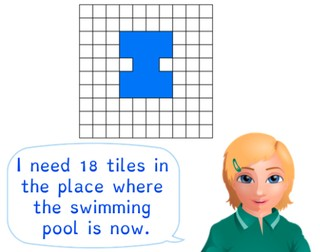Determining area using squares

# Determining area using squares

Students learn to determine area using squares.

No account needed.8,000 schools use Gynzy92,000 teachers use Gynzy1,600,000 students use Gynzy

## General

The students learn how they can determine an area using squares.

## Standards

CCSS.Math.Content.3.MD.C.5/6

## Learning objective

Students will be able to determine an area using squares.

## Introduction

You show the shapes on the interactive whiteboard and ask what the area of each shape is. After the students have given an answer, you can ask how they determined this. After that you can erase the cover to show what the correct answer is.

## Instruction

Explain that the area is the size of the surface of a shape. You can determine how large the area is using different methods. First discuss this with a shape with straight lines. You can count the colored squares. You can also use the multiplication tables. For this you do the length × the width. If the shape is not a square or a rectangle, then you have included too many squares in your calculation. You subtract these extra squares from your total. Another method is to divide the shape into squares. If you know how many small squares are in the larger square, then you can multiply this by the number of big squares in the shape. Then you add the leftover (small) squares to your total. You can also determine the area of a shape with diagonal lines. If the lines go exactly through the middle of squares then those are half squares. You add the half squares together to make whole squares. Have the students practice determining the area of shapes with diagonal lines. After this you show a shape in which the diagonal lines do not run exactly through the middle of the squares. Explain that you can divide this shape up in pieces and match the pieces together, to make whole squares. The students then practice determining the area of this type of shape.

To check whether the students understanding how they can determine area, you can ask the following question:
- How do you determine the area [of this shape]?

## Quiz

The students test their understanding of determining area using squares through ten exercises. In each of the exercises they must determine the area of a shape. Some of these shapes are irregular, and some include diagonal lines.

## Closing

You discuss once again with the students how it is importnat to be able to determine area using squares, because that is how you can quickly calculate the area of a shape. Show three shapes and ask which of the shapes has an area of 27. After that, you show a different shape for which the students must determine the area. There are possible answers listed that the students can choose from.

## Teaching tips

When students have difficulty determining area using squares, have them draw a line through the shape, to help keep track of the squares that they have counted in the shape. After that they can practice multiplying length and width.

### The online teaching platform for interactive whiteboards and displays in schools

• Save time building lessons

• Manage the classroom more efficiently

• Increase student engagement